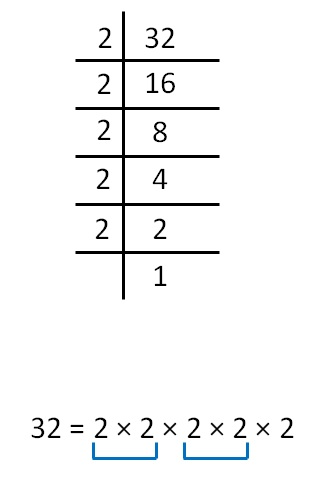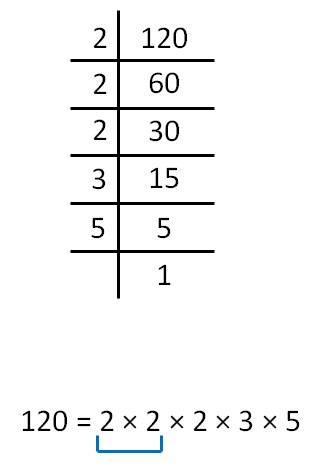Checking if perfect square by prime factorisation

Chapter 5 Class 8 Squares and Square Roots
Concept wise

Here,

• We do prime factorization of the number
• If the prime factorization forms pairs, then it is a perfect square
• If it doesn’t, then it isn't a perfect square

Let’s take some examples

#### Is 32 a perfect square?Here, we do not get perfect square as one 2 is left alone.

So, 32 is not a perfect square

#### Is 120 a perfect square?Here, 2, 3, 5 are not in pairs

So, 120 is not a perfect square

Learn in your speed, with individual attention - Teachoo Maths 1-on-1 Class